# Plus Two Accountancy Chapter Wise Questions and Answers Chapter 3 Use of Spread Sheet in Business Application

Students can Download Chapter 3 Use of Spread Sheet in Business Application Questions and Answers, Plus Two Accountancy Chapter Wise Questions and Answers helps you to revise the complete Kerala State Syllabus and score more marks in your examinations.

## Kerala Plus Two Chemistry Chapter Wise Questions and Answers Chapter 3 Use of Spread Sheet in Business Application

Question 1.
Which of the following options in a financial function indicates the interest for a period?
(a) FV
(b) PV
(c) N per
(d) Rate
(b) PV

Question 2.
Which of the following arguments in a financial function represents the total number of payments?
(a) FV
(b) PV
(c) N Per
(d) Rate
(c) N-PerQuestion 3.
What Category of functions is used in this formula: = PMT(C 10/12, C8, C9, 1)
(a) Logical
(b) Financial
(c) Payment
(d) Statistical
(b) Financial

Question 4.
LibreOffice Calc is a program
(a) Word Processor
(b) Browser
(d) Calculator

Question 5.
……………… is the statement prepared to show detailed salary calculation
(a) Employee Job Card
(b) Payroll
(c) Loan Repayment Schedule
(d) Worksheet Payroll
(b) payrollQuestion 6.
………………… calculates the monthly installment of loan amount
(a) Loan Repayment Schedule
(b) Loan analysis sheet
(c) Loan card
(d) Payroll statement
(a) Loan Repayment schedule

Question 7.
Depreciation is provided on
(a) Current Assets
(b) Fixed Assets
(c) Current Liabilities
(d) Long term Liabilities
(b) Fixqd Assets

Question 8.
Depreciation =
$$\frac{\text { cost of the asset- Scrap Value }}{\text { Life of the asset }}$$

Question 9.
……………………. is the gradual & permanent diminution in the value of assets due to wear and tear, use or abuse or efflux of time.
Depreciation

Question 10.
Under …………… method of depreciation, the asset account will be reduced to zero.
(a) Fixed Instalment method
(b) Reducing installment
(c) Depreciation Fund Method
(d) Revaluation method
(a) Fixed Instalment MethodQuestion 11.
Decrease in the value of fixed assets is called ……………..
Depreciation

Question 12.
If the cost of asset is 10000, Scrap value at the end of 10 years will be 2000, what will be the amount of annual depreciation?
Depreciation = $$\frac{10000-2000}{10}$$ i.e., 800

Question 13.
Depreciation = (Acquisition cost – ………………. ) ÷ Life time
(a) Salvage value
(b) Carriage expenses
(c) Sales price
(d) Installation charges
(a) Salvage value

Question 14.
The ……… of an asset is the value, which is realisable at the end of its useful life
(a) Depreciation
(b) Scrap value
(c) Written down value
(d) Acquisition cost
(b) Scrap value

Question 15.
Odd one out
(a) Basic Pay
(c) House Rent Allowance
(d) Provident Fund
(d) Provident Fund (It is a deduction)

Question 16.
_________ is a statutory deduction deducted monthly towards income tax liability of an employee
Tax Deducted at Source (TDS)

Question 17.
Gross Salary – Total Deduction = ______
Net SalaryQuestion 18.
In PMT function, Type is, whether payment is made at the beginning of the month, the value = ……(a)…… or at the end of the month, the value = …….(b)……
(a) 1
(b) 0

Question 19.
Rate of Depreciation under straight line Method = ………
$$\frac{\text { Amount of Depreciation (Yearty Depreciation) }}{\text { Total Depreciable Amount (Cost) }} \times 100$$

Question 20.
The function PMT is used to prepare …………….
(a) Pay Roll statement
(b) Depreciation statement
(c) Loan repayment statement
(d) Interest on Investment statement
(c) Loan repayment statement

Question 21.
Match the following

 A B (1) SLN (a) Written down value method of depreciation (2) DB (b) Monthly salary statement (3) Salvage value (c) Fixed Instalment method of depreciation (4) Payroll (d) Acquisition Cost – Total Depreciation

1 → c; 2 → a; 3 → d; 4 → b

Question 22.
Which among the following is not a component of PayRoll statement
(a) Professional Tax
(b) Present Value
(c) HRA
(d) Dearness Allowance
(b) Present Value

Question 1.
What are the different methods for calculating depreciation on fixed Assets?
Methods of calculation of depreciation

1. Straight Line Method (SLM)
2. Written Down Value Method (WDV)

Question 2.
What commands are used to

1. Insert a column and
2. Delete a column in Libre Office Calc

1. Insert a column
2. Delete a columnQuestion 3.
Develop the command to calculate the Group Insurance Premium and Tax Deducted at source (TDS) by using the ‘IF’ function.

1. Rate of GI Rs. 200/-. for BP below Rs. 10,000/- and for others Rs. 300/- assuming that BP of employee is given in cell F2
2. TDS 10% of Gross Pay for employees having Gross Pay below Rs, 25000/- and for others 20%, assuming that the gross pay of the employee is given in cell F2.

1. = IF (B2 < 10000,200,300)
2. = IF (F2 < 25000, F2*10%, F2*20%)

Question 4.
Name the two basic method of depreciation functions used in LibreOffice Calc

1. SLN
2. D8

Question 5.
Why is FV taken as Zero (0) in the PMT calculation?
At the end of the loan period, the balance amount payable will be zero assuming that the repayments are made on regular basis. Therefore the future value FV is taken as zero.

Question 6.
Write the command to calculate the State Life Insurance (SLI) Premium of an Employee using the ‘IF’ function.
The condition is:- SLI Premium Rs. 250 for basic pay below Rs. 10,000/- for others Rs.500/- (Hint: Basic pay (BP) is given the cell B3)
IF (B3 < 10000, 250, 500)

Question 1.
Give some examples for PayRoll components.

1. Basic Pay
2. Dearness Allowance
3. House Rent Allowance
4. Provident Fund
5. Professional Tax
6. ESI

Question 2.
Basic Pay, Dearness Allowance, House Rent allowance, Professional Tax, Provident fund contribution are given to prepare the PayRoll statement. Give the equation to calculate Net Salary.

• Gross salary = Basic Pay + Dearness Allowance + House Rent Allowance
• Total Deduction = Professional Tax + Provident fund contribution
• Net Salary = Gross Salary – Total Deduction

Net Salary Calculation:
Step 1 – Calculate Gross salary by using the given formula.
Gross salary /Gross Pay = Basic Pay + Grade Pay + Dearness Pay+ Dearness Allowance + House Rent Allowance + Any other Earnings.

Step 2 – Calculate Total Deduction by using the following formula.
Total Deduction = Professional Tax+ Provident Fund + Tax deducted at source + Loan Recovery + Any other deductions

Step 3- Calculate net salary by the given formula.
Net Salary = Gross salary – Total DeductionQuestion 3.
What is the difference between WDV method and SLN method of depreciation?
Written Down Value Method (WDV):
This method is also known as Diminishing balance method or Reducing balance method. Under this method, a fixed percentage is written off every year on the book value of the asset at the beginning of the year.

Here the amount of depreciation goes on decreasing and therefore, the book value of asset will not become zero after its working life.
Amount of depreciation = Written Down Value of asset x Rate of depreciation

Straight Line Method:
Under this method a fixed amount is deducted from the value of an asset year after year on account of depreciation and debited to profit and loss account. This method is also called Fixed Instalment method, or Original Cost method. Under this method value of asset will be reduced to zero.
Depreciation = $$\frac{\text { cost of the asset-Scrap Value }}{\text { Life of the asset }}$$

Question 4.
List down the Parameters of the function PMT
LOAN REPAYMENT SCHEDULE:
Loan is a sum of borrowed money for a specified period at a pre-specified rate of interest. The loan is repaid through a number of periodic repayment instalments over the loan repayment period. LibreOffice Calc function PMT is used to calculate the loan repayment schedule. The parameters of the function PMT are as follows.

Parameter – Explanation

• Rate – Interest rate
• Nper – Total Number of payments for the loan
• PV – Present value(Loan amount)
• FV – Future value, which is taken a zero, is the balance at the end of the loan period
• Type – Whether payment is made at the beginning (value = 1) or at the end (value = 0) of the period.

Question 5.
Classify the assets under computerised asset accounting.
Assets are classified into the following categories:

1. Goodwill
2. Land: Freehold and leasehold
3. Building: Factory, office & residential building
4. Plant & Machinery
5. Furniture and fixtures
6. Vehicles
7. Work in progress (Capital)
8. Other assetsQuestion 6.
List out common Payroll components regarding salary computation and its payment.
Earnings:

1. Basic pay
2. DearnessAllowances
3. House Rent Allowances
4. Transport Allowances
5. Other allowances.

Deductions:

• Provident Fund
• Professional Tax
• Tax deducted at source

Question 7.
What are the common accounting applications done with the help of Libre Office Calc?

1. Payroll Accounting
2. Asset Management
3. Loan Repayment Schedule

Question 8.
Write the formula in Libre Office Calc to find the Professional Tax in cell B2 where annual income is given in cell A2. Profession Tax is 5% for income in between Rs. 100000 and Rs.200000 and 8% for income more than Rs. 2,00,000. No tax for income below Rs. 1,00,000.
= IF(B2 > 200000, B2*8%, IF(B2>100000, B2*5%,))

Question 1.
Write command to calculate state life Insurance Premium (SLI) of employee using the ‘IF’ function.
Condition
Premium Rs. 350/- below Basic Pay of Rs. 25000 and for others Rs. 450/- (BP is given in cell A3)
= IF(A3 < 25000, 350, 450)Question 2.
Briefly explain the procedure of preparation of salary bill and disbursement of cash.
Preparation of Salary Bill:
The preparation of salary bill should provide for the following:
1. Maintaining payroll related data such as Employee No., Name, attendance, Basic Pay, DA, and other allowances, deductions to be made etc.

2. Periodic Payroll Computations:
It includes the calculation of various earnings and deductions.

3. Preparation of salary statement and employee’s salary slip.

4. Generation of advice to bank:
It contains the net salary to be transferred to individual bank account of employees and other salary related statutory payments such as provident fund, tax, etc.

Question 3.
The column headings of payroll to be prepared through Libre Office Calc is given below.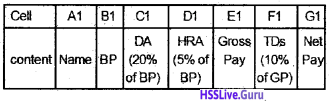1. Write the formula to calculate DA, HRA, Gross pay, TDS and Net pay of Jexin Jose the first employee, in the second row of the worksheet.
2. Give the command to fill the calculation automatically for the remaining ‘10’ employees in the firm.

1. DA → C2 =B2 * 20%
HRA → D2 = B2 * 5%
GP → E2 = B2 + C2+ D2
TDS → F2 = E2 * 10%
NP → G2 = E2 – F2

2. Fill the calculation for the remaining ‘10’ employees in the firm.

Question 4.
Distinguish between straight line method and diminishing balance method of depreciation

 Straight line Method Diminishing balance Method 1. A Fixed amount is deducted from the value of an asset. 1. The amount of depreciation goes on reducing year after year. 2. Depreciation is computed on the original cost of the asset. 2. Depreciation is calculated on the written down value of the asset. 3. The value of the asset is reduced to zero at the end of effective working life. 3. The value of the asset will not become zero after its effective working life. 4. The method is also known as Fixed Instalment method or original cost method. 4. This method is also known as reducing balance method or written down value method.Question 5.
Mr. Jyothis, a Plus two commerce student, entered the following details in a worksheet of LibreOffice Calc.Write the command to calculate Net SalaryFill down the calculation to remaining 4 employees

Question 6.
Develop the commands to calculate the group insurance premium (Gl) and Tax Deducted at source (TDS) by using the ‘IF’ function.

1. Rate of GI Rs. 200/- for BP below Rs: 8000/- and for others Rs. 300/-, assuming that BP of employee is given in B2.
2. TDS 10% of Gross pay, for employees having Gross pay below Rs. 15,000/- and for others, 20%, assuming that the gross pay of the employee is given in F2.

1. IF (B2 < 8000, 200, 300)
2. IF (F2 < 15000, F2*10%, F2*20%)

Question 1.
How the assets are classified in computerised Asset Accounting? What are the different methods of calculating depreciation on such assets?
In computerised Asset Accounting, Assets are classified into the following categories.

1. Goodwill
2. Land (Normally, depreciation is not provided on freehold land)
3. Building
4. Plant and Machinery
5. Furniture and Fixtures
6. Vehicles
7. Work in progress (Capital)
8. Others

The different methods of calculating depreciation are:

• Straight Line Method (SLM)
• Written Down Value Method (WDV)Question 2.
Which built in function of LibreOffice Calc can be used to compute monthly instalments of repayment of loan? Give the parameters of this function.
PMT function can be used to prepare Loan Repayment Schedule in LibreOffice Calc.
The parameters of PMT function are:-

Parameter – Explanation

• Rate – Interest on Loan
• Nper – Number of payments for the loan
• PV – Present value; (ie the loan amount)
• FV – Future value, which is taken a zero
• Type – If the payment is made at the beginning of the month, the value = 1 or at the end of the month, the value = 0

Question 3.
From the following particulars prepare a payroll of employees of a firm by using LibreOffice Calc

• DA – 40% of basic pay
• HRA – 8% of basic pay
• Contribution to PF – 10% of basic pay.

• DA = Basic pay *40%
• HRA = Basic pay *8%
• PF = Basic pay *10%
• Gross Pay = Basic Pay + DA + HRA
• Net Pay = Gross pay – PF

Question 4.
From the following particulars, prepare a payroll of employees of a firm by using LibreOffice Calc.

• DA = 70% of Basic pay
• HRA = 10% of Basic pay
• Contributions to PF at 15% of Basic pay.

Step 1 – Enter the following

 Cell Content A1 Name of employee B1 Basic pay C1 DA D1 HRA E1 GROSS SALARY F1 PF G1 Net Salary

Step 2

 Cell Formula C2 = B1 * 70% D2 = B1 * 10% E2 = B2 + C2 + D2 F2 = B1 * 15% G2 = E2 – F2

Step 3 – Copy this formula to the remaining cells

Question 1.
Prepare a Pay Roll statement of Viswanath Enterprises from the table given below and additional information.1. DA is provided at 90% of Basic Pay
2. HRA: Rs. 500 for manager, 400 for accountant and 200 for others.
3. PF is deducted @ 20% on Basic + DAProcedure:
Step 1 – Open a blank worksheet in LibreOffice Calc.

Step 2 – Enter the following text/formula in respective cellsStep 3 – Enter the given details

 Cell Formula E2 = D2 * 90% F2 = IF(C2 = “Manager”, 500, IF(C2 = “Accountant”, 400, 200)) G2 = SUM (D2: F2) H2 = SUM(D2: E2) * 20% K2 = SUM(H2: J2) L2 = G2 – K2

Step 4 – Copy the formula down up to the last employee.
Output: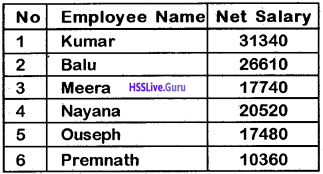Question 2.
Mr. Shibu wants to take a housing loan of Rs. 2,00,000 repayable in 60 equal monthly installments over the next 5 years. Assuming that the installments are paid in the beginning of each month. Find out the amount of monthly installments. Use PMT Function.
Procedure:
Step 1 – Open blank work sheet in LibreOffice Calc.
Applications → Office → Libre Office Calc.

Step 2 – Enter the following data in appropriate cellsOutput:

 Monthly Installments -4,404.84Question 3.
Riya took a industrial loan of Rs. 300000, repayable in 4 years (equal monthly installments). The annual rate of interest is 10%. Assuming that the installments are paid at the end of each month. Find out the amount of monthly installments. Use PMT Function.
Procedure:
Step 1 – Open a blank work sheet in LibreOffice Calc.
Application → Office → Libre Office Calc.

Step 2 – Enter the following data in appropriate cells.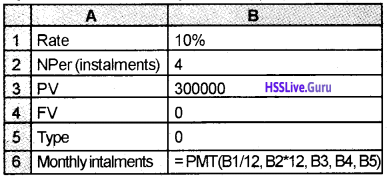Output:

 Monthly Installments -7608.78

Question 4.
From the following particulars of employees in Anu Traders, prepare the Pay Roll.

 Name Basic pay Thomson 8760 Nilson 9340 Shibu 10100 Shijo 7690 Shyjan 8350 Rejo 11200

1. DA – 50% of BP
2. HRA – 10% of BP
3. A monthly subscription to PF – 15% of BP
4. Group insurance premium -120 from each employee.

Procedure:
Step 1 – Open a new worksheet in LibreOffice Calc

Step 2 – Enter the following details in the following cells.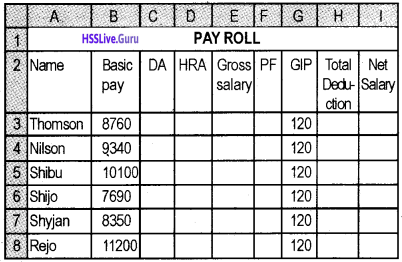Step 3 – Enter the following details in the respective cells

 Cell Formula C3 = B3 * 50% D3 = B3 * 10% E3 = SUM(B3: D3) F3 = B3 * 15% H3 = F3 + G3 13 = E3 – H3

Step 4 – Copy the formula down up to the last employee.
Output:

 Name Net Salary Thomson 12582 Nilson 13423 Shibu 14525 Shijo 11030.50 Shyjan 11987.50 Rejo 16120

Question 5.
The salary information of a company named Seasons India Ltd. is given below. Prepare Payroll.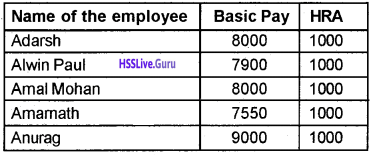DA – 50% of Basic pay, CCA – 5% of Basic pay, PF 8% of Basic pay.
Procedure:
Step 1 – Open a blank worksheet in LibreOffice Calc

Step 2 – Enter the following details in the following cells.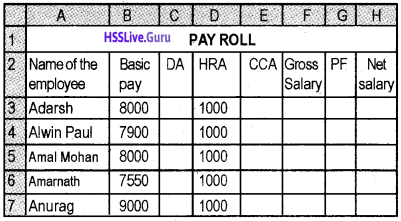Step 3 – Enter the following formula in respective cells

 Cell Formula C2 = B3 * 50% E2 = B3 * 5% F2 = SUM(B3: E3) G2 = B3 * 8% H2 = F3 – G3

Step 4 – Copy the formula down up to the last employee
Output:

 Name of Employee Net Salary Adarsh 12760 Alwin Paul 12613 Amal Mohan 12760 Amarnath 12098.50 Anurag 14230Question 6.
Mr.Anil Kumar a plus two commerce student entered the following details in a worksheet of LibreOffice Calc.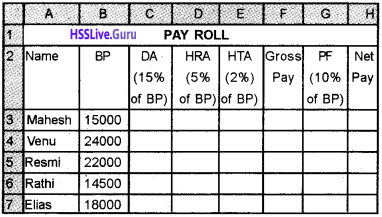But, he faces some difficulty in completing the task. Can you help him by giving necessary commands or formula for filling the bank columns in the given spread sheet.
Give the Following Formula:

 Cell Formula C3 = B3 * 15% D3 = B3 * 5% E3 = B3 * 2% F3 = B3 + C3 + D3 + E3 G3 = B3 * 10% H3 = F3 – G3

Copy these formula to the remaining cell. Edit – Fill – Down or use Drag option.
Output: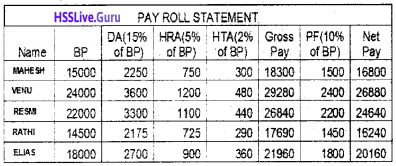Question 7.
Prepare the payroll of Amal Bros, for the month of January 2016.• DA – 36% of Basic Pay
• HRA – Rs 500
• CCA – 6% of Basic pay

Deduction:

1. PF subscription – 8% of BP.
2. Group insurance premium Rs. 200 below basic pay Rs. 6000 and for others Rs. 350.
3. Tax deducted at source 10% of Gross Pay for employees below gross pay of Rs. 10000 arid for others 20%. Also, find out the total salary payable to employees for the month.

Procedure:
Step 1 – Open a blank work sheet in LibreOffice Calc

Step 2 – Enter the following details in the following cells.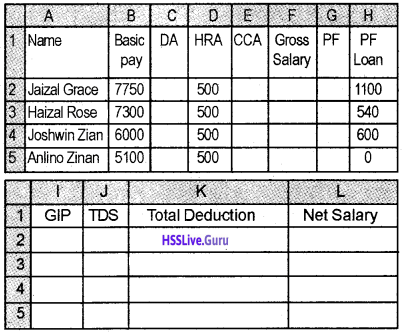Step 3 – Enter the following formula in the respective cell

 Cell Formula C2 = B2 * 36% E2 = B2 * 6% F2 = SUM(B2: E2) G2 = B2 * 8% 12 = IF(B2 < 6000, 200, 350) J2 = IF(F2 < 10000, F2 * 10%, F2 * 20%) K2 = SUM(G2: J2) L2 = F2 – K2

Step 4 – Copy the formula down up to the last employee.
Output:

 Name of Employee Net Salary Jaizal Grace 7134 Haizal Rose 7218.80 Joshwin Zian 6688 Anlino Zinan 6359.80Question 8.
Prepare a pay roll of Jayakumar Associates for the month of June 2016 from the following details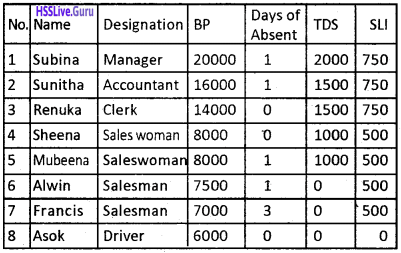1. DA – 50% of Basic pay earned
2. HRA – Manager – 30%, Accountant – 20%, clerk 10%, no HRA for saleswoman/Salesman and driver.
3. Transport allowance – 1500 for saleswoman, 2500 for salesman and 1000 for driver
4. PF contribution – 6% of BP for all employees except the driver.

Procedure:
Step 1 – Open a blank worksheet in LibreOffice Calc

Step 2 – Enter the following text/formula in the following way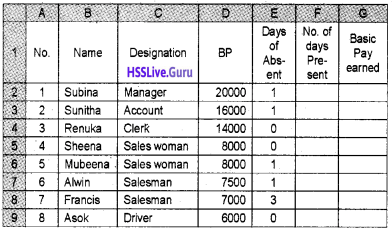Step 3 – Enter the given formula in F2
F2 = 30 – E2
Copy the formula down to the last employee

Step 4 – Enter the given formula in G2
G2 = D2*F2 ÷ 30
Copy the formula down to the last employee.

Step 5 – Enter the text for formula in the following cells.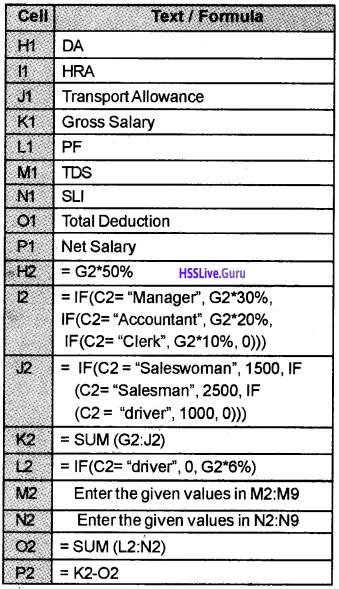Step 6 – Copy the formula down to the last employee.
Output: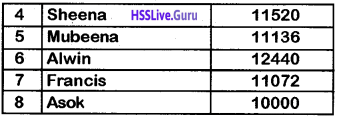Question 9.
Megha Associates purchased a new Machinery on 1/1/2010 for Rs. 8000 and spent Rs. 2000 for its installation. The expected salvage value is Rs. 2000 at the end of its useful life of 10 years. Calculate the amount of depreciation under straight line method.
Procedure:
Step 1 – Open a new blank worksheet in LibreOffice Calc.

Step 2 – Enter the following details as follows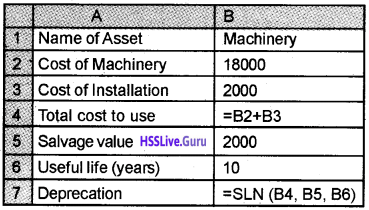Output:

 Depreciation 1800

Question 10.
On 1.1.2010, Vahida Enterprises purchased a new machinery for Rs. 150000 and incurred Rs. 10000 for its installation. Preoperative expense amounted to Rs. 5000. The expected salvage value at the end of its useful life of 8 years is Rs. 2000. Calculate depreciation by straight line method by using spread sheet.
Step 1 – Open a new blank worksheet in LibreOffice Calc.

Step 2 – Enter the following details in respective cells.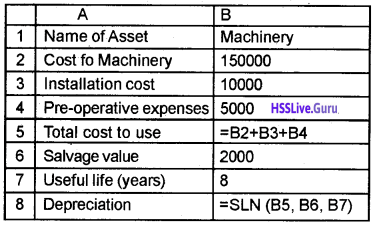Output:

 Depreciation 20375

Question 11.
On 1st April 2000, a company purchased a Machinery for Rs. 20,00,000, The installation charge is 50,000, pre-operation expenses are 1,50,000 and its salvage value is calculated Rs. 1, 00,000. Life of machinery is estimated to 15 years. Calculate depreciation under straight line method.
Procedure:
Step 1 – Open a new blank worksheet in LibreOffice Calc.

Step 2 – Enter the following data/formula in the following way.Output:

 Depreciation 1,40,000Question 12.
From the following details, calculate depreciation under written down value method by using spread sheet.

1. Purchase fo Machinery – 10-6-2012
2. Cost of the Machinery – 300000
3. Machinery installed on – 15-6-2012
4. Installation Expenses – 2000
5. Pre operating cost – 13000
6. Salvage value after 8 years – 18000
7. 1st year end date – 31/3/2013

Procedure:
Step 1 – Open a new blank worksheet in LibreOffice Calc.

Step 2 – Enter the given data/ formula as follows.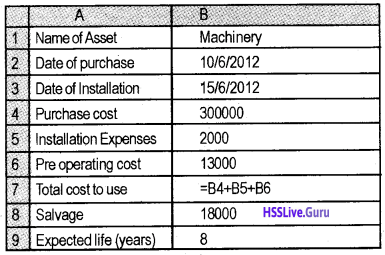Output:

 Depreciation 79012.5

Question 13.
From the following details, calculate depreciation of machinery under written down value method using spreadsheet.

 Name of asset Machinery Date of purchase 10-5-2009 Date of installation 20-5-2009 Purchase cost 200000 Installation cost 30000 Pre operating cost 20000 Salvage value 10,000 Expected life of asset 8 years 1st year end date 31/3/2010

Step 1 – Open a new blank worksheet in LibreOffice Calc.

Step 2 – Enter the given data in respective cells.Output:

 Depreciation 75854.2Question 14.
From the following details, calculate depreciation underwritten down value Method.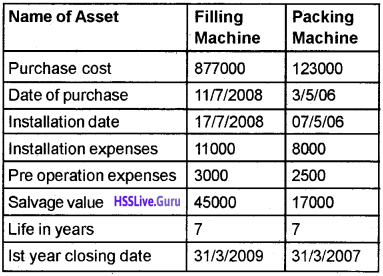Procedure:
Step 1 – Open a new blank worksheet in LibreOffice Calc.

Step 2 – Enter the following data in the respective cells.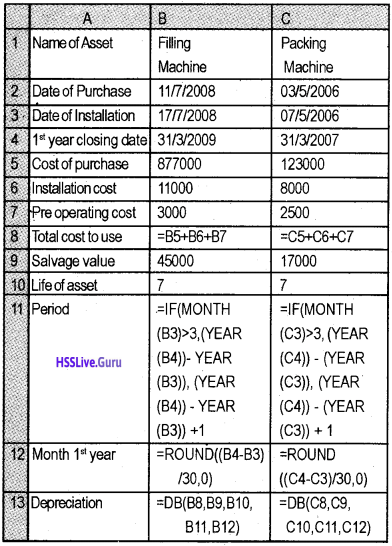Output:

 Depreciation – Filling Machine 2,31,882.75 Depreciation – Packing Machine 31,205.63

Question 15.
On 1-2-2015, Vikram borrowed Rs. 6,00,000 from Canara bank at 9.6% interest. The period of loan is 36 months. Calculate monthly installment assuming the installments are made at the beginning of each month.
procedure:
Step 1 – Open a new blank worksheet in LibreOffice Calc.

Step 2 – Enter data/formula as follows: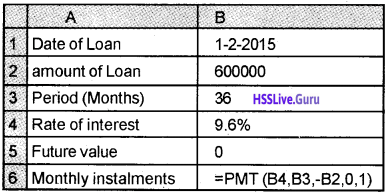Output:

 Monthly Loan installment 54567.3Question 16.
Mr. Chandramohan has taken a loan of Rs. 300,000 from a bank at an interest rate of 10% p.a. The loan is to be repaid in 36 monthly installments over the next 3 years. Assuming that the monthly installments are paid at the end of each month. Calculate the amount of interest paid by him in the 2nd year only. Use CUMIPMT Function.
Procedure:
Step 1 – Open a new blank worksheet in LibreOffice Calc.

Step 2 – Enter the following details and formula in different cells as given below.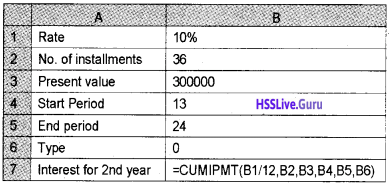Output:

 Interest for the 2nd year 16491.6